# 9.6 SPM Practice (Long Questions)

Question 9 (12 marks):
A(25o N, 35o E), B(25o N, 40o W), C and D are four points which lie on the surface of the earth. AD is the diameter of the common parallel latitude 25o N .
(a) Find the longitude of D.

(b)
C lies 3300 nautical miles due south of measured along the surface of the earth.
Calculate the latitude of C.

(c)
Calculate the shortest distance, in nautical mile, from to measured along the surface of the earth.

(d)
An aeroplane took off from C and flew due north to point A.
The total time taken for the whole flight was 12 hours 24 minutes.

(i)
Calculate the distance, in nautical mile, from A due west to measured along the common parallel of latitude.

(ii)
Calculate the average speed, in knot, of the whole flight.

Solution:
(a)
Longitude of D = (180o – 35o)W
= 145oW

(b)

(c)
Shortest distance of to D
= (65o + 65o) × 60’
= 130o × 60’
= 7800 nautical miles

(d)(i)
Distance from A to B
= (35o + 40o) × 60’ × cos 25o
= 75o × 60’ × cos 25o
= 4078.4 nautical miles

(d)(ii)

# 9.6 SPM Practice (Long Questions)

Question 8 (12 marks):
Diagram 8 shows four points, G, H, I and J on the surface of the Earth. JI is the diameter of the parallel of latitude 50o N. O is the centre of the Earth.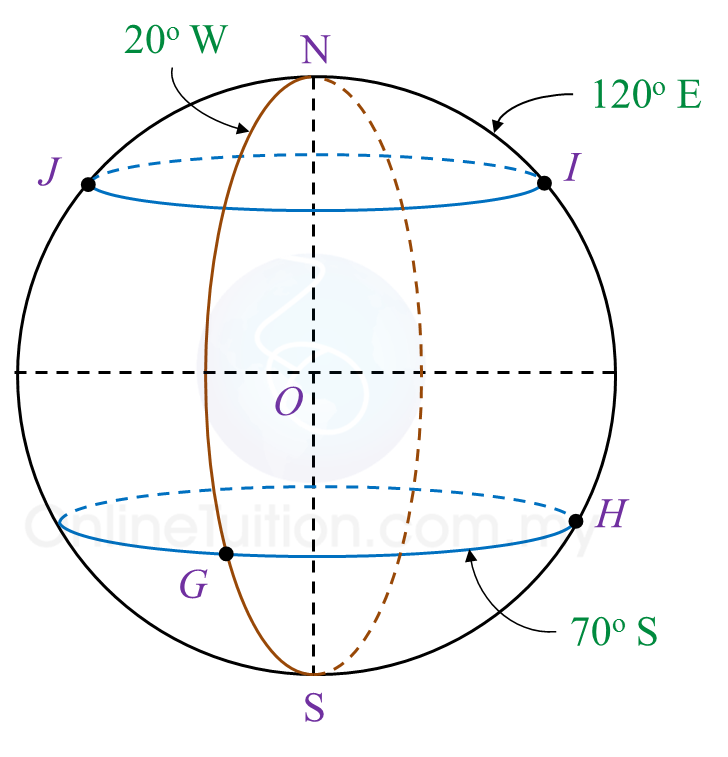Diagram 8

(a)
State the location of G.

(b) Calculate the shortest distance, in nautical mile, from I to J measured along the surface of the Earth.

(c) Calculate the shortest distance, in nautical mile, from G to H measured along the common parallel of latitude.

(d) An aeroplane took off from I and flew due south to point P. The average speed of the journey was 800 knots. The time taken for the flight was 5.25 hours.
Calculate the latitude of P.

Solution:
(a)
Location of G = (70o S, 20o W)

(b)
∠ JOI
= 180o – 50o – 50o
= 80o
Distance of I to J
= 80o × 60’
= 4800 nautical miles

(c)
Distance of G to H
= (20o + 120o) × 60’ × cos 70o
= 140o × 60’ × cos 70o
= 2872.97 nautical miles

(d)

# 9.6 SPM Practice (Long Questions)

Question 7 (12 marks):
Diagram 7 in the answer space shows the locations of points J, L and M, which lie on the surface of the earth. O is the centre of the earth. The longitude of M is 30o W. K is another point on the surface of the earth such that KJ is the diameter of the common parallel of latitude 45o S.

(a)(i)
Mark and label point K on Diagram 7 in the answer space.

(ii)
Hence, state the longitude of point K.

(b)
L lies due north of M and the shortest distance from M to L measured along the surface of the earth is 7500 nautical miles.
Calculate the latitude of L.

(c)
Calculate the distance, in nautical mile, from K due east to M measured along the common parallel of latitude.

(d)
An aeroplane took off from K and flew due east to M along the common parallel of latitude. The average speed of the aeroplane for the flight was 750 knots.
Calculate the total time, in hour, taken for the whole flight.Solution:
(a)(i)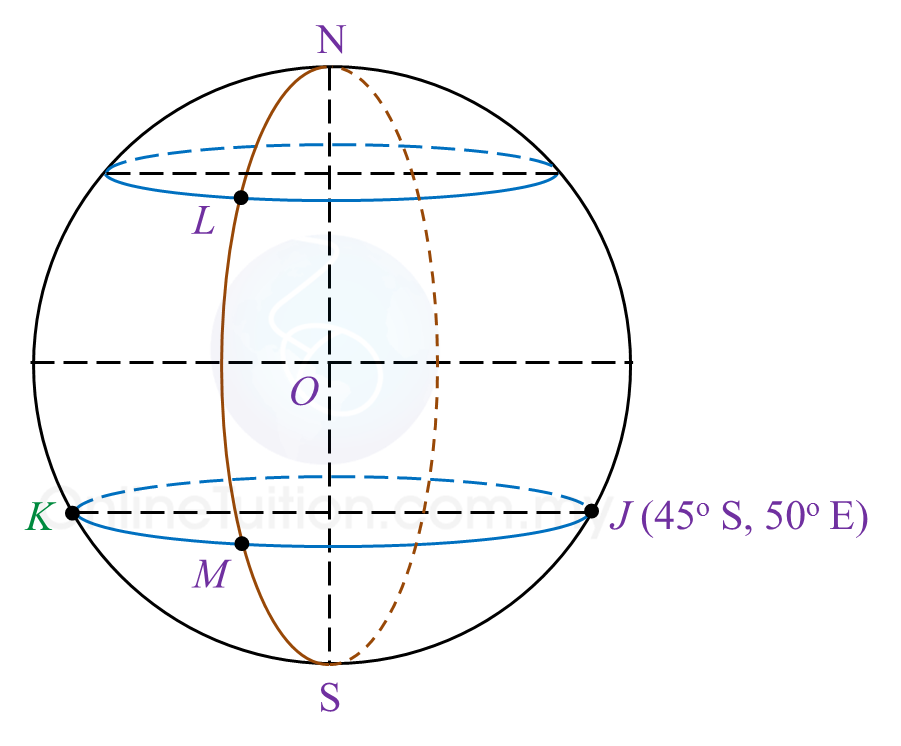(a)(ii)
Longitude of point K = 130oW

(b)

(c)
KM = (130o – 30o) × 60 × cos 45o
= 4242.64 nautical miles

(d)

# 9.6 SPM Practice (Long Questions)

Question 6:
Diagram below shows the locations of points P, Q, R, A, K and C, on the surface of the earth. O is the centre of the earth.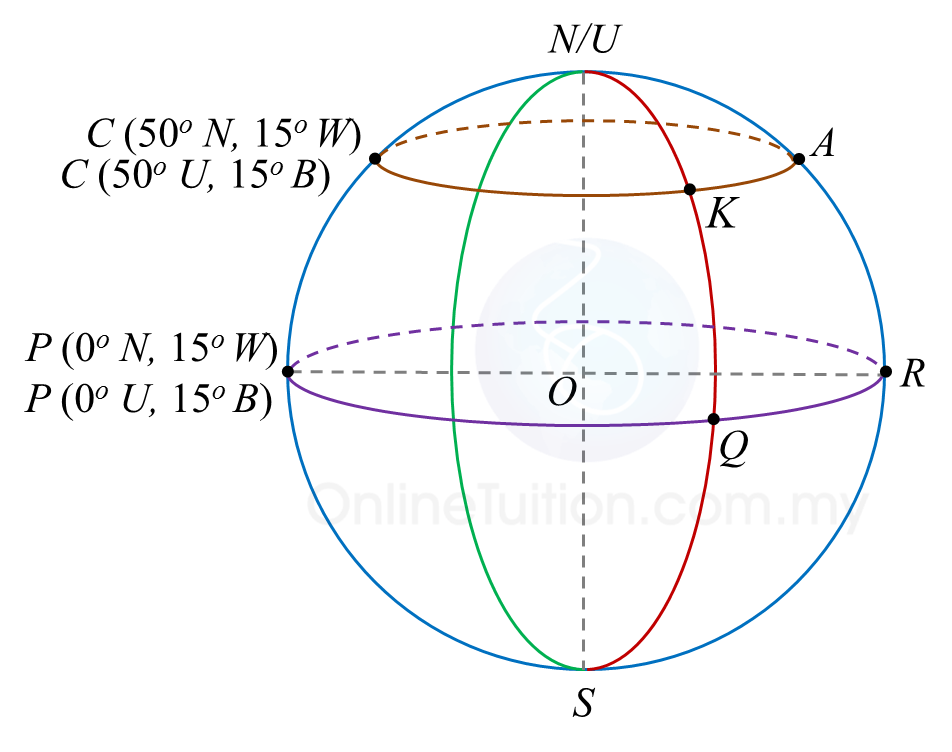(a) Find the location of A.

(b) Given the distance QR is 3240 nautical miles, find the longitude of Q.

(c) Calculate the distance, in nautical miles of KA, measured along the common parallel latitude.

(d) An aeroplane took off from A and flew due west to K along the common parallel of latitude. Then, it flew due south to Q. The average speed of the aeroplane was 550 knots.
Calculate the total time, in hours, taken for the whole flight.

Solution:
(a)
Longitude of A = (180o – 15o) = 165o E
Latitude of A = 50o N
Therefore, position of A = (50o N, 165o E).

(b)

(c)
Distance of KA
= 54 x 60 x cos 50o
= 2082.6 nautical miles

(d)

# 9.6 SPM Practice (Long Questions)

Question 5:
A (53o N, 84o E), B (53o N, 25o W), C and D are four points on the surface of the earth. AC is the diameter of the parallel of latitude 53o N.

(a)
State the location of C.

(b)
Calculate the shortest distance, in nautical mile, from A to C measured along the surface of the earth.

(c)
Calculate the distance, in nautical mile, from A due east B measured along the common parallel of latitude.

(d)
An aeroplane took off from B and flew due south to D. The average speed of the flight was 420 knots and the time taken was 6½ hours.
Calculate
(i) the distance, in nautical mile, from B to D measured along the meridian.
(ii) the latitude of D.

Solution:
(a)
Latitude of C = 53o N
Longitude of C = (180o – 84o) E = 96o E
Therefore location of C = (53o N, 96o E)

(b)
Shortest distance from A to C
= (180 – 53 – 53) x 60
= 74 x 60
= 4440 nautical miles

(c)
Distance from A to B
= (84 – 25) x 60 x cos 53o
= 59 x 60 x cos 53o
= 2130.43 nautical miles

(d)

# 9.6 SPM Practice (Long Questions)

Question 4:
P (25o N, 35o E), Q (25o N, 40o W), R and S are four points on the surface of the earth. PS is the diameter of the common parallel of latitude 25o N.

(a) Find the longitude of S.

(b) R lies 3300 nautical miles due south of P measured along the surface of the earth.
Calculate the latitude of R.

(c) Calculate the shortest distance, in nautical mile, from P to S measured along the surface of the earth.

(d) An aeroplane took off from R and flew due north to P. Then, it flew due west to Q.
The total time taken for the whole flight was 12 hours 24 minutes.

(i) Calculate the distance, in nautical mile, from P due west Q measured along the common parallel of latitude.
(ii) Calculate the average speed, in knot, of the whole flight.

Solution:
(a)
Longitude of S = (180o – 35o) W = 145o W

(b)

(c)
Shortest distance from P to S
= (65 + 65) x 60
= 130 x 60
= 7800 nautical miles

(d)(i)
Distance of PQ
= (35 + 40) x 60 x cos 25o
= 75 x 60 x cos 25o
= 4078.4 nautical miles

# 9.6 SPM Practice (Long Questions)

Question 3:
Diagram below shows the locations of points A (34o S, 40o W) and B (34o S, 80o E) which lie on the surface of the earth. AC is a diameter of the common parallel of latitude 34o S.(a) State the longitude of C.

(b) Calculate the distance, in nautical mile, from A due east to B, measured along the common parallel of latitude 34o S.

(c) K lies due north of A and the shortest distance from A to K measured along the surface of the earth is 4440 nautical miles.
Calculate the latitude of K.

(d) An aeroplane took off from B and flew due west to A along the common parallel of latitude. Then, it flew due north to K. The average speed for the whole flight was 450 knots.
Calculate the total time, in hours, taken for the whole flight.

Solution:
(a)
Longitude of C = (180o – 40o) E = 140o E

(b)
Distance of AB
= (40 + 80) x 60 x cos 34o
= 120 x 60 x cos 34o
= 5969 nautical miles

(c)

(d)

# 9.6 SPM Practice (Long Questions)

Question 1:
Diagram below shows four points P, Q, R and M, on the surface of the earth. lies on longitude of 70oW. QR is the diameter of the parallel of latitude of 40o N. M lies 5700 nautical miles due south of P.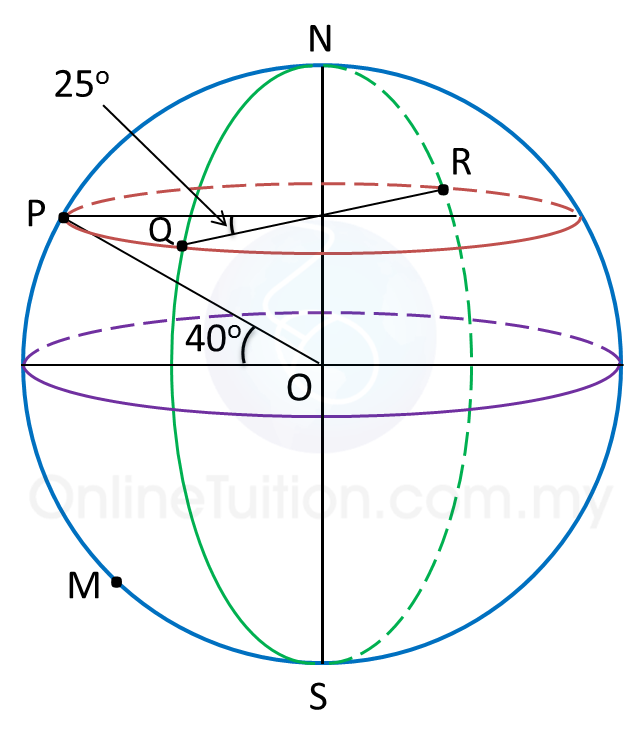(a) Find the position of R.

(b)
Calculate the shortest distance, in nautical miles, from Q to R, measured along the surface of the earth.

(c)
Find the latitude of M.

(d)
An aeroplane took off from R and flew due west to P along the parallel of latitude with an average speed of 660 knots.

Calculate the time, in hours, taken for the flight.

Solution:
(a)
Latitude of = latitude of Q = 40o N
Longitude of = (70o – 25o) W = 45o W
Longitude of = (180o – 45o) E = 135o E
Therefore, position of R = (40o N, 135oE).

(b)
Shortest distance from Q to R
= (180 – 40 – 40) x 60
= 100 × 60
= 6000 nautical miles

(c)
$\begin{array}{l}\angle POM=\frac{5700}{60}\\ \text{}={95}^{o}\\ \therefore \text{Latitude of}M=\left({95}^{o}-{40}^{o}\right)S\\ \text{}={55}^{o}S\end{array}$

(d)
$\begin{array}{l}\text{Time taken =}\frac{\text{distance from}R\text{to}P}{\text{average speed}}\\ \text{}=\frac{\left(180-25\right)×60×\mathrm{cos}{40}^{o}}{660}\\ \text{}=\frac{155×60×\mathrm{cos}{40}^{o}}{660}\\ \text{}=10.79\text{hours}\end{array}$

# 9.6 SPM Practice (Long Questions)

Question 2:
P(25o S, 40o E), Qo N, 40o E), R(25o S, 10o W) and are four points on the surface of the earth. PK is the diameter of the earth.

(a)
State the location of point K.

(b)
Q is 2220 nautical miles from P, measured along the same meridian.
Calculate the value of θ.

(c)
Calculate the distance, in nautical mile, from P due west to R, measured along the common parallel of latitude.

(d)
An aeroplane took off from Q and flew due south to P. Then, it flew due west to R. The average speed of the aeroplane was 600 knots.
Calculate the total time, in hours, taken for the whole flight.

Solution:(a)
As PK is the diameter of the earth, therefore latitude of K = 25o N
Longitude of K= (180o – 40o) W = 140o W
Therefore, location of K = (25o N, 140oW).

(b)
Let the centre of the earth be O.
$\begin{array}{l}\angle POQ=\frac{2220}{60}\\ \text{}={37}^{o}\\ {\theta }^{o}={37}^{o}-{25}^{o}={12}^{o}\\ \therefore \text{The value of}\theta \text{is 12}\text{.}\end{array}$

(c)
Distance from to R
= (40 + 10) × 60 × cos 25o
= 50 × 60 × cos 25o
= 2718.92 n.m.

(d)
Total distance travelled
= distance from to P + distance from P to R
= 2220 + 2718.92
= 4938.92 nautical miles
$\begin{array}{l}\text{Time taken =}\frac{\text{total distance from}Q\text{to}R}{\text{average speed}}\\ \text{}=\frac{4938.92}{600}\\ \text{}=8.23\text{hours}\end{array}$

# 9.5 SPM Practice (Short Questions)

Question 1
In diagram below, N is the North Pole and is the South Pole. The location of point is (40o S, 70o W) and POQ is the diameter of the earth.Find the longitude of Q.

Solution:
Since PQ is a diameter of the earth and the longitude of P is θo W, the longitude of Q is (180o – θo) E.
Longitude of = 70o W
Longitude of = (180o – 70o) E
= 110oE

Question 2
In diagram below, N is the North Pole and is the South Pole and NOS is the axis of the earth.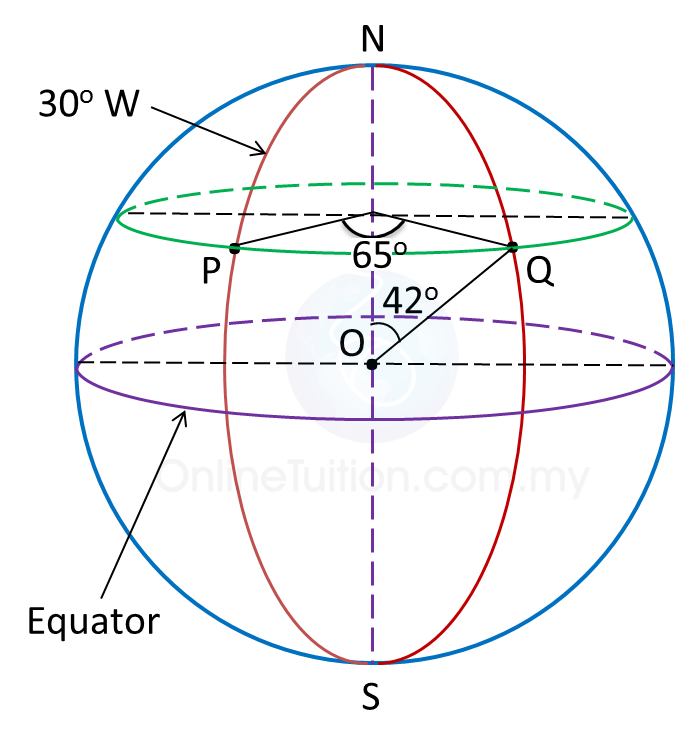Find the position of point Q.

Solution:
Latitude of = (90o – 42o) N
= 48o N
Longitude of = (65o – 30o) E
= 35o E
Therefore, position of Q = (48o N, 35oE).